# Gnat-power sawtooth oscillator works on low supply voltages

## TS12011 TS12012

Bruce D Moore

EDN

This sawtooth-oscillator circuit, drawing less than 3.2 μA and working at under 1 V, is a useful building block that fits the bill for extremely low-power consumption and operation to low supply voltages. It could be used as the basis for a PWM control loop, a timer, or a VCO, or as a capacitance-to-frequency converter. It’s a nifty circuit for two reasons: It uses an open-drain comparator output to make an accurate switched current source, and it uses a latch function to make a simple comparator into a window comparator, while needing no extra components.

The appeal of this circuit is found in the combination of the tiny size, the ridiculously low number of external components, a low supply current, and the ability to maintain a constant amplitude and frequency despite the variable battery voltage. Unlike the classic op-amp astable multivibrator, this design features comparator thresholds that are set by precision reference voltages rather than the output swing of the op amp in combination with resistor feedback.

A ratiometric fixed-frequency design of this type usually results in a variable-amplitude sawtooth waveform, which is undesirable in PWM control loops because it can affect the loop gain. As a side benefit, the up/down ramps can be independently controlled by scaling R1 and R2.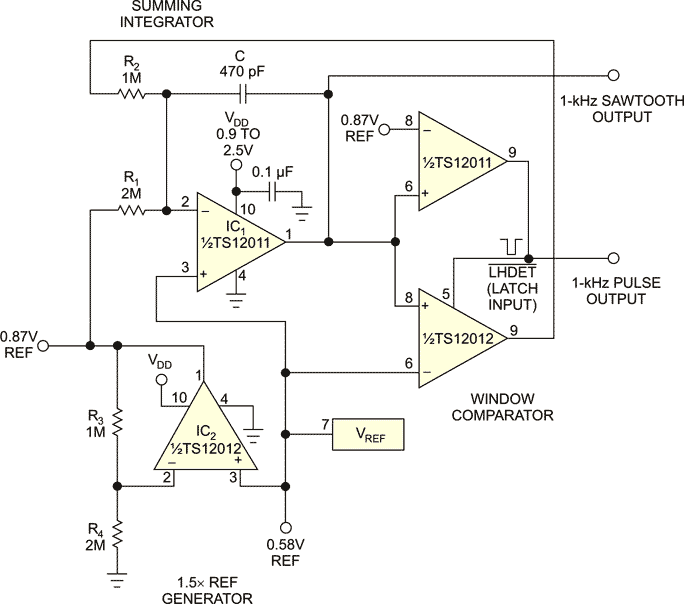Figure 1. This low-voltage sawtooth generator uses only eight components and draws extremely low power.

Referring to Figure 1, there are only eight components in this circuit: two ICs, four resistors, a capacitor, and a power-supply-bypass capacitor. The key bits are two Touchstone Semiconductor analog building-block ICs in 4-mm2 TDFN packages (the TS12011 and the TS12012), each containing an op amp, a comparator, and a reference (Figure 2). By leaning on their characteristics, the design can be kept terrifically tiny and simple.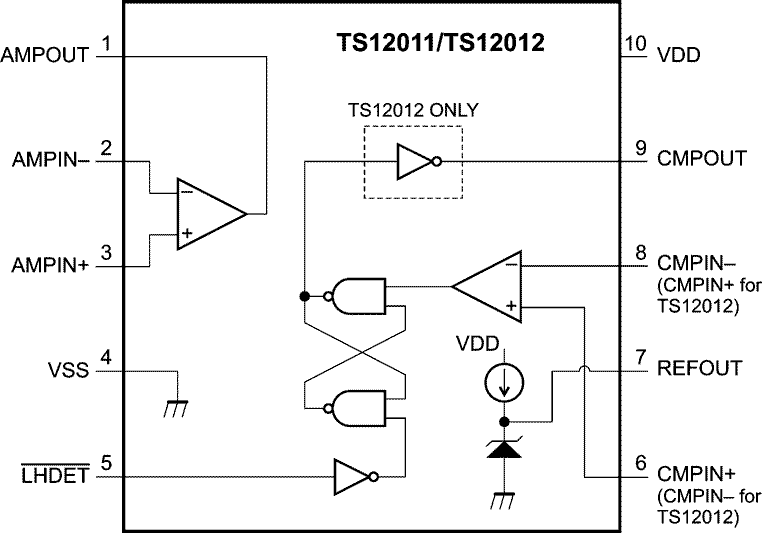Figure 2. TS12011/TS12012 Block Diagram.

Here’s how the circuit works: A summing integrator feeding a window comparator generates the sawtooth wave. The integrator-summing node is held at VREF by the feedback action of the amplifier. Thus, a fixed positive reference current set by R1 is balanced by a larger-amplitude switched negative current set by R2. The lower comparator block has an open-drain output; when its output is low, current is pulled from the summing node via R2: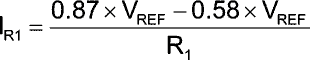and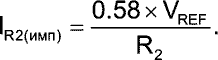If IR2 is set to 2×IR1, a symmetrical triangle wave results.

The frequency is set as follows: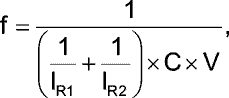where V is the difference between 0.87×VREF and 0.58×VREF. Here, f=850 Hz.

Figure 3 shows the waveforms at the sawtooth and pulse outputs.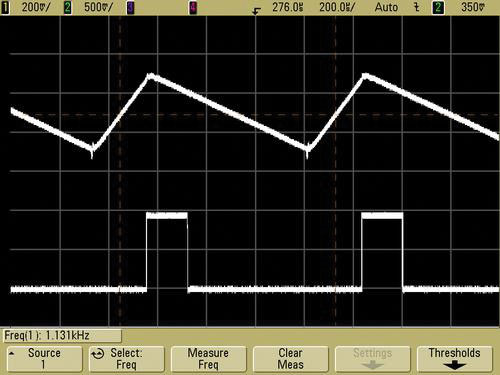Figure 3. The waveforms at the sawtooth and pulse outputs are shown. The pulse train is used to reset the latching comparator.

The window comparator employs a built-in latch function of the TS12012 to provide hysteresis. The latch function has a sly feature: When /LHDET is pulled low, the comparator inputs are still active and sensing the input state, until the inputs cross. The comparator in IC2 gets set when the ramp crosses the lower threshold at 0.58×VREF, and reset when the ramp crosses 0.87×VREF. The reset pulse is momentary, but puts the latch in a state where the comparator inputs crossing cause it to set and latch again (which happens due to the switched reference current causing the integrator to ramp negative). The net result: No glue logic is needed.

The battery voltage ranges down to 0.9 V with a miserly VDD current of 3.2 μA. Maximum operating frequency is limited by the op-amp slew rate and prop delays to about 3 kHz. Disconnecting R1 and driving it with a voltage source greater than 0.58×VREF gives you a VCO function.

EDN